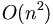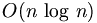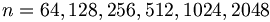Fast Fourier Transform

(diff) ← Older revision | Latest revision (diff) | Newer revision → (diff)
Fast Fourier transform (FFT) is an efficient algorithm for calculating the discrete fourier transform (DFT). The FFT produces the same results as a DFT but it reduces the execution time by hundreds in some cases. Whereas DFT takes an order of$O(n^2)\,$ computations, FFT takes an order of$O(n\,\log\,n)$, and is definitely the preferred algorithm to be used in all applications in terms of computational complexity. The FFT in most implementations consistent of samples that are exactly a power of 2, this is commonly known as a FFT Radix 2 algorithm where$n = 64,128,256,512,1024,2048$ etc.